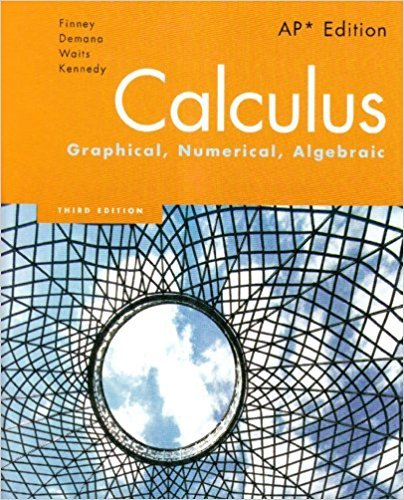×
×

# Solutions for Chapter 2: Calculus: Graphical, Numerical, Algebraic 3rd Edition## Full solutions for Calculus: Graphical, Numerical, Algebraic | 3rd Edition

ISBN: 9780132014083Solutions for Chapter 2

Solutions for Chapter 2
4 5 0 413 Reviews
20
4
##### ISBN: 9780132014083

This expansive textbook survival guide covers the following chapters and their solutions. This textbook survival guide was created for the textbook: Calculus: Graphical, Numerical, Algebraic, edition: 3. Since 55 problems in chapter 2 have been answered, more than 4518 students have viewed full step-by-step solutions from this chapter. Chapter 2 includes 55 full step-by-step solutions. Calculus: Graphical, Numerical, Algebraic was written by and is associated to the ISBN: 9780132014083.

Key Calculus Terms and definitions covered in this textbook
• equation of a quadratic function

ƒ(x) = ax 2 + bx + c(a ? 0)

• Expanded form of a series

A series written explicitly as a sum of terms (not in summation notation).

• Inverse tangent function

The function y = tan-1 x

• Linear equation in x

An equation that can be written in the form ax + b = 0, where a and b are real numbers and a Z 0

• Linear regression line

The line for which the sum of the squares of the residuals is the smallest possible

• Logarithm

An expression of the form logb x (see Logarithmic function)

• Magnitude of a real number

See Absolute value of a real number

• Matrix element

Any of the real numbers in a matrix

• Midpoint (in Cartesian space)

For the line segment with endpoints (x 1, y1, z 1) and (x2, y2, z2), ax 1 + x 22 ,y1 + y22 ,z 1 + z 22 b

• NDER ƒ(a)

See Numerical derivative of ƒ at x = a.

• Proportional

See Power function

A function that can be written in the form ƒ(x) = ax 2 + bx + c, where a, b, and c are real numbers, and a ? 0.

The measure of an angle in radians, or, for a central angle, the ratio of the length of the intercepted arc tothe radius of the circle.

• Re-expression of data

A transformation of a data set.

• Reciprocal of a real number

See Multiplicative inverse of a real number.

• Scientific notation

A positive number written as c x 10m, where 1 ? c < 10 and m is an integer.

• Shrink of factor c

A transformation of a graph obtained by multiplying all the x-coordinates (horizontal shrink) by the constant 1/c or all of the y-coordinates (vertical shrink) by the constant c, 0 < c < 1.

• Trigonometric form of a complex number

r(cos ? + i sin ?)

• Vertical asymptote

The line x = a is a vertical asymptote of the graph of the function ƒ if limx:a+ ƒ1x2 = q or lim x:a- ƒ1x2 = q.

• Vertical translation

A shift of a graph up or down.

×